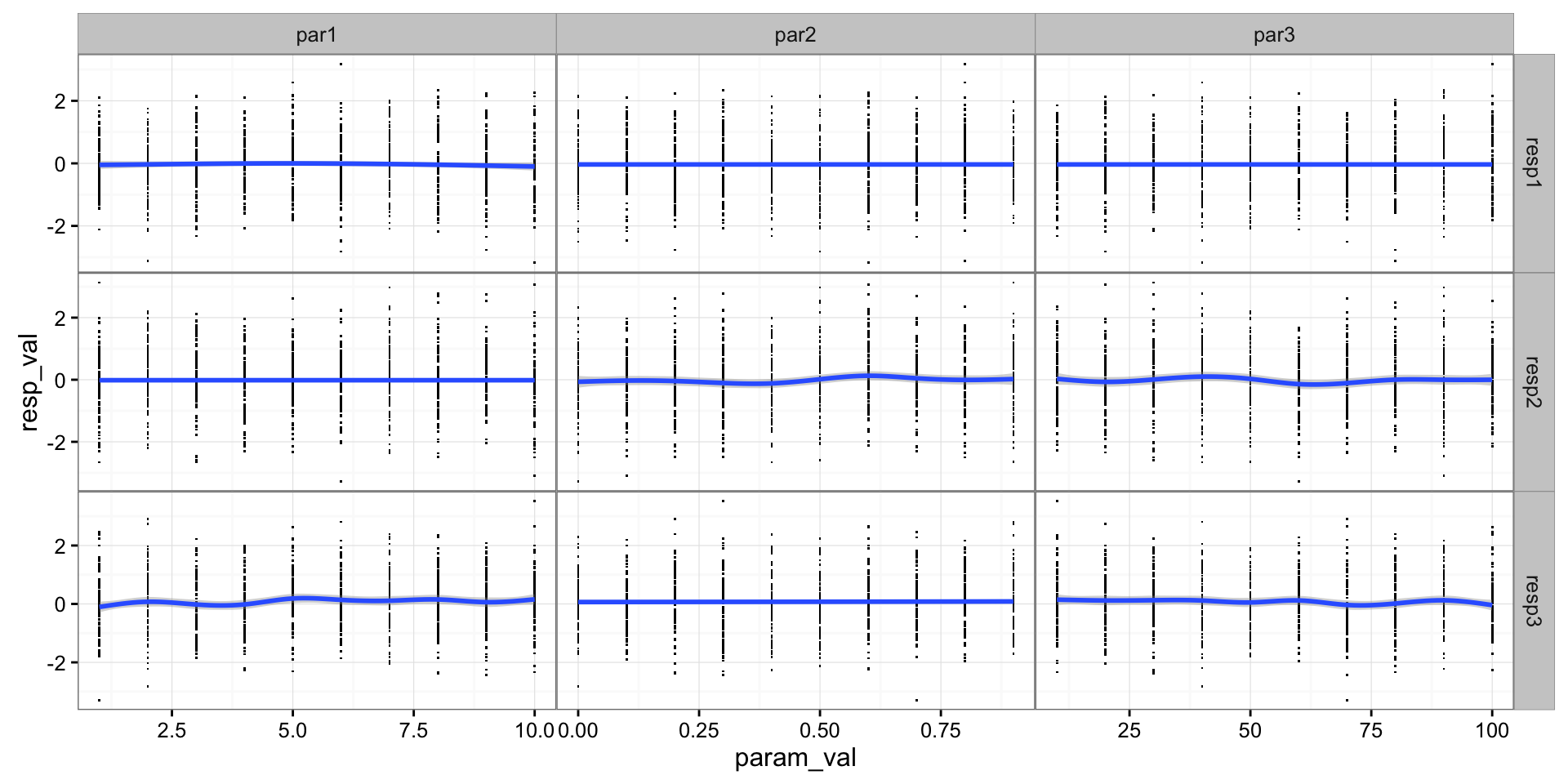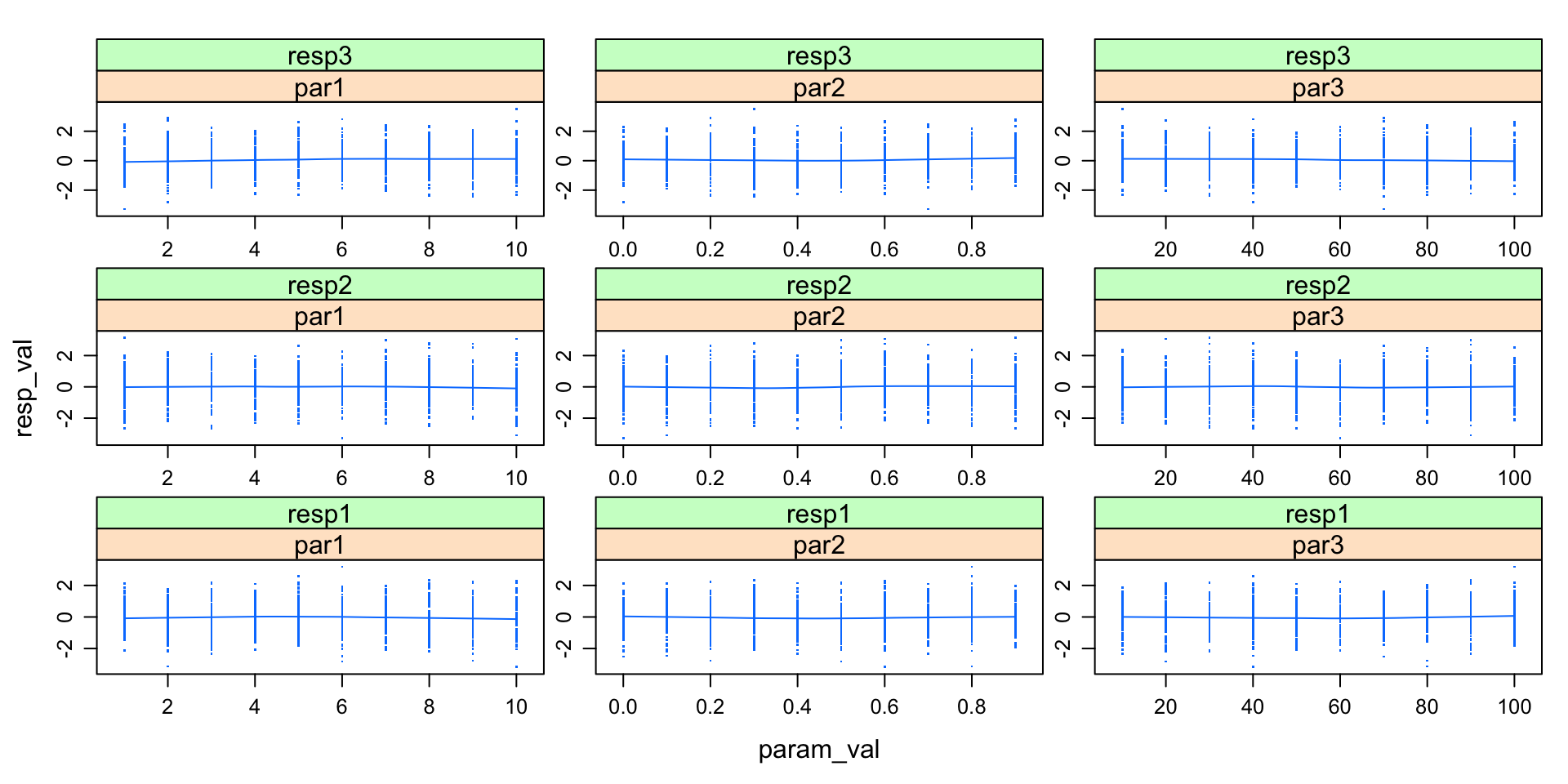library(reshape2)
library(dplyr)
library(tidyr)
library(tibble)
library(ggplot2); theme_set(theme_bw())

# Starting from an array

Suppose I have an array of results from a 3-way factorial experiment; each run had three different response variables. (If the results for your simulation runs are stored in a long data frame with the parameters and response variables for each run in a single row, skip to the next section.)

set.seed(101)
a0 <- array(rnorm(3000),
dim=c(10,10,10,3),
dimnames=list(par1=1:10,par2=seq(0,0.9,0.1),par3=(1:10)*10,
var=c("resp1","resp2","resp3")))
## melt() automatically converts dimnames to numeric unless as.is=TRUE

The first step is to melt, i.e. convert the 10x10x10x3 array to a 4x3000 data frame (actually, a tbl_df, pronounced “tibble”: tibbles are enhanced/modified data frames, a part of Hadley Wickham’s “tidyverse”)

am0 <- melt(a0) %>% as_tibble
print(am0,n=3)
## # A tibble: 3,000 × 5
##    par1  par2  par3    var      value
##   <int> <dbl> <int> <fctr>      <dbl>
## 1     1     0    10  resp1 -0.3260365
## 2     2     0    10  resp1  0.5524619
## 3     3     0    10  resp1 -0.6749438
## # ... with 2,997 more rows

Add an identifier variable for later merging:

am0$run <- seq(nrow(am0))  Pull out the part of the data set with the response variables (which is already in long or “melted” format): resp_dat <- am0 %>% select(run,var,value) %>% rename(resp=var,resp_val=value) print(resp_dat,n=3) ## # A tibble: 3,000 × 3 ## run resp resp_val ## <int> <fctr> <dbl> ## 1 1 resp1 -0.3260365 ## 2 2 resp1 0.5524619 ## 3 3 resp1 -0.6749438 ## # ... with 2,997 more rows Pull out the part of the data set with the parameter values and convert it to long form: select_ is needed when we want to use a character vector to specify column names. param_names <- names(dimnames(a0))[1:3] param_dat <- am0 %>% select_(.dots=c("run",param_names)) %>% gather(key=param,value=param_val,-run) ## wide to long print(param_dat,n=3) ## # A tibble: 9,000 × 3 ## run param param_val ## <int> <chr> <dbl> ## 1 1 par1 1 ## 2 2 par1 2 ## 3 3 par1 3 ## # ... with 8,997 more rows Now we can combine the parameter and response variables. comb_dat <- full_join(resp_dat,param_dat,by="run") print(comb_dat,n=3) ## # A tibble: 9,000 × 5 ## run resp resp_val param param_val ## <int> <fctr> <dbl> <chr> <dbl> ## 1 1 resp1 -0.3260365 par1 1 ## 2 1 resp1 -0.3260365 par2 0 ## 3 1 resp1 -0.3260365 par3 10 ## # ... with 8,997 more rows If you’re not curious about other data formats, you can skip to the last section (on plotting). # Starting from a data frame of parameters and responses Alternatively you might have data that looks like this, with one line per run containing both the parameter and response values: (e.g. from a Latin hypercube sample): dd <- dcast(am0,par1+par2+par3~var) %>% as_tibble print(dd,n=3) ## # A tibble: 1,000 × 6 ## par1 par2 par3 resp1 resp2 resp3 ## <int> <dbl> <int> <dbl> <dbl> <dbl> ## 1 1 0 10 -0.3260365 0.5031958 1.1643810 ## 2 1 0 20 0.2680658 -0.7536373 -0.2503754 ## 3 1 0 30 -0.1640324 1.1731488 -1.2111637 ## # ... with 997 more rows The only difference from the previous workflow is that now we treat the parameter and response variables symmetrically - both need to be selected and converted to long form: dd$run <- seq(nrow(dd)) ## add identifier variables
## convert parameter values to long format
resp_names <- paste0("resp",1:3)
param_dat <- dd %>%
select_(.dots=c("run",param_names)) %>%
gather(key=param,value=param_val,-run)
resp_dat <- dd %>%
select_(.dots=c("run",resp_names)) %>%
gather(key=resp,value=resp_val,-run)
comb_dat <- full_join(resp_dat,param_dat,by="run")

# Sensitivity plot

I normally use ggplot for these plots; it makes it easy if you want to add other information (colours by groups, different point sizes, etc.).

## for squashing panels together
zero_margin <- theme(panel.margin=grid::unit(0,"lines"))
ggplot(comb_dat,aes(param_val,resp_val))+
geom_point(pch=".")+   ## pch="." -> minimal point size
facet_grid(resp~param,scale="free")+
geom_smooth()+
zero_marginOne advantage of the older lattice graphics system is that it renders complex plots considerably faster - but it is a bit more difficult to customize.

lattice::xyplot(resp_val~param_val|param*resp,
data=comb_dat,pch=".",
type=c("p","smooth"),
scales=list(relation="free"))